# Multiplication - 7th grade (12y) - math problems

#### Number of problems found: 181

• In centerWhat number lies in the center between a quarter of a fifth and a half of a third on the number axis?
• No. of divisorsHow many different divisors have number ??
• Number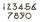What number I think? The third is 6 and half is 2 more than the its quarter.
• Division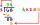Divide by the number 0.2 is the same as multiply by what number?
• Brackets 2Add parenthesis to make true: 5-2×6-4+2=5
• Cube 3How many times will increase the volume of a cube if we double the length of its edge?
• Coin and die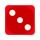Flip a coin and then roll a six-sided die. How many possible combinations are there?
• LCMWhat is the least common multiple of 5, 50, 14?
• Babysitting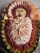\$27.40 babysitting for 4 hours. How much would she make if she babysat for 7 hours?
• Twenty-fiveHow many three-digit natural numbers are divisible by 25?
• Percent calculationCalculate 51% if 99% is 79.
• Telephone numbers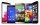How many 7-digit telephone numbers can we put together so that each number consists of different digits?
• Expressions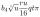Expression (3a-7).(3a+7) is identical with: ?
• Cube 2How many times will increase if the surface area of the cube if we triple length of its edge?
• SymbolsIf 2*3 = 60 ; 3*4 = 120 and 4*5 = 200, what is 2*5?
• Factors 2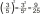A number has 3 identical factors. If 1 of them is 18. Find the number step by step
• Two thirdsFind two-thirds of the number equal to two-thirds of 99
• Discount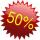Product has been discounted twice by 19%. What is the total discount given?
• Percent chaining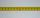Calculate 20% from 70% from 80 km
• Colors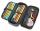Willie got birthday 6 colour pens in different colors. How many ways he can give them side by side in pencil?

Do you have an interesting mathematical word problem that you can't solve it? Submit a math problem, and we can try to solve it.

We will send a solution to your e-mail address. Solved examples are also published here. Please enter the e-mail correctly and check whether you don't have a full mailbox.

Please do not submit problems from current active competitions such as Mathematical Olympiad, correspondence seminars etc...